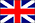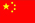Hi, welcome to Dongguan Haoda Electronic Co., Ltd.!
EnglishChineseproduct center
4NEWS CENTER

Inductance coil main characteristic parameter

Inductance coil main characteristic parameter
1, inductance L inductance L said the inherent characteristics of the coil itself, regardless of the size of the current.In addition to a special inductor coil (color code inductor), the inductance is generally not specifically marked on the coil, and a specific name.
2, inductance XL inductance coil of the AC current blocking effect of the size of said inductive reactance XL, in ohms, and its inductance L and AC frequency f relationship for the XL = 2πfL.
3, the quality factor Q quality factor Q is a physical quantity that coil quality, Q is the inductance XL and its equivalent resistance ratio, namely: Q = XL / R coil Q value is higher, the smaller the loop loss. Q value of the wire is related to the DC resistance of the wire, the dielectric loss of the frame, the loss due to the shield or the core, the influence of the high frequency skin effect, etc. The Q value of the coil is usually several tens to several hundreds.
4, the distributed capacitance coil between the turns and turns, between the coil and the shield, the coil and the bottom plate between the existence of the capacitance is called the distributed capacitance of the existence of distributed capacitance of the coil Q value decreases, the stability of deterioration, and coil The smaller the distributed capacitance, the better.